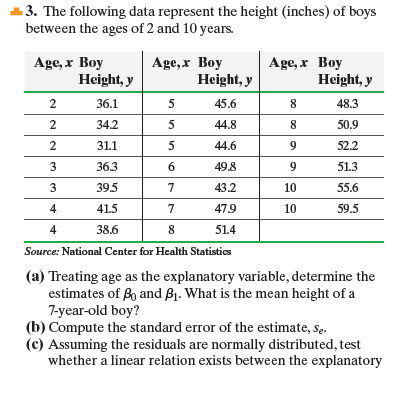×
Get Full Access to Statistics: Informed Decisions Using Data - 5 Edition - Chapter 14 - Problem 3
Get Full Access to Statistics: Informed Decisions Using Data - 5 Edition - Chapter 14 - Problem 3

×

# ?The following data represent the height (inches) of boys between the ages of 2 and 10 years. (a) Treating age as the explanatory variable, deISBN: 9780134133539 240

## Solution for problem 3 Chapter 14

Statistics: Informed Decisions Using Data | 5th Edition

• Textbook Solutions
• 2901 Step-by-step solutions solved by professors and subject experts
• Get 24/7 help from StudySoup virtual teaching assistantsStatistics: Informed Decisions Using Data | 5th Edition

4 5 1 397 Reviews
29
4
Problem 3

The following data represent the height (inches) of boys between the ages of 2 and 10 years.

(a) Treating age as the explanatory variable, determine the estimates of b0 and b1. What is the mean height of a 7-year-old boy?

(b) Compute the standard error of the estimate, se.

(c) Assuming the residuals are normally distributed, test whether a linear relation exists between the explanatory variable, age, and response variable, height, at the= 0.05 level of significance.

(d) Assuming the residuals are normally distributed, construct a 95% confidence interval for the slope of the true leastsquares regression line.

(e) Construct a 90% confidence interval for the mean height found in part (a).

(f) Predict the height of a 7-year-old boy.

(g) Construct a 90% prediction interval for the height found in part (f).

(h) Explain why the predicted heights found in parts (a) and (f) are the same, yet the intervals are different.Step-by-Step Solution:

Step 1 of 5) The following data represent the height (inches) of boys between the ages of 2 and 10 years. (a) Treating age as the explanatory variable, determine the estimates of b0 and b1. What is the mean height of a 7-year-old boy (b) Compute the standard error of the estimate, se. (c) Assuming the residuals are normally distributed, test whether a linear relation exists between the explanatory variable, age, and response variable, height, at the = 0.05 level of significance. (d) Assuming the residuals are normally distributed, construct a 95% confidence interval for the slope of the true leastsquares regression line. (e) Construct a 90% confidence interval for the mean height found in part (a). (f) Predict the height of a 7-year-old boy. (g) Construct a 90% prediction interval for the height found in part (f). (h) Explain why the predicted heights found in parts (a) and (f) are the same, yet the intervals are different.

Step 2 of 1

##### ISBN: 9780134133539

The full step-by-step solution to problem: 3 from chapter: 14 was answered by , our top Statistics solution expert on 01/15/18, 03:19PM. Statistics: Informed Decisions Using Data was written by and is associated to the ISBN: 9780134133539. This full solution covers the following key subjects: . This expansive textbook survival guide covers 88 chapters, and 2422 solutions. This textbook survival guide was created for the textbook: Statistics: Informed Decisions Using Data, edition: 5. The answer to “?The following data represent the height (inches) of boys between the ages of 2 and 10 years.(a) Treating age as the explanatory variable, determine the estimates of b0 and b1. What is the mean height of a 7-year-old boy?(b) Compute the standard error of the estimate, se.(c) Assuming the residuals are normally distributed, test whether a linear relation exists between the explanatory variable, age, and response variable, height, at the = 0.05 level of significance.(d) Assuming the residuals are normally distributed, construct a 95% confidence interval for the slope of the true leastsquares regression line.(e) Construct a 90% confidence interval for the mean height found in part (a).(f) Predict the height of a 7-year-old boy.(g) Construct a 90% prediction interval for the height found in part (f).(h) Explain why the predicted heights found in parts (a) and (f) are the same, yet the intervals are different.” is broken down into a number of easy to follow steps, and 146 words. Since the solution to 3 from 14 chapter was answered, more than 234 students have viewed the full step-by-step answer.

## Discover and learn what students are asking

Statistics: Informed Decisions Using Data : The Binomial Probability Distribution
?State the criteria for a binomial probability experiment.

Statistics: Informed Decisions Using Data : The Normal Approximation to the Binomial Probability Distribution
?In Problems 5–14, a discrete random variable is given. Assume the probability of the random variable will be approximated using the normal distributio

Statistics: Informed Decisions Using Data : The Normal Approximation to the Binomial Probability Distribution
?In Problems 5–14, a discrete random variable is given. Assume the probability of the random variable will be approximated using the normal distributio

Unlock Textbook Solution